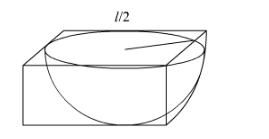# A hemispherical depression is cut out from one face of a cubical`
Question:

A hemispherical depression is cut out from one face of a cubical wooden block such that the diameter 'l' of the hemisphere is equal to the edge of the cube. Determine the surface area of the remaining solid.

Solution:

It is given that a hemisphere is cut from a cubical box with edge such that

diameter of hemisphere is also l.

We have to find the surface area of the remaining solid.Surface area of the cubical box with side $l=6 l^{2}$

Let $r$ be the radius of hemisphere

Surface area of the hemisphere $=\pi r^{2}+2 \pi r^{2}$

$=\pi\left(\frac{l}{2}\right)^{2}+2 \pi\left(\frac{l}{2}\right)^{2}\left(\right.$ since $\left.r=\frac{l}{2}\right)$

Surface area of the remaining solid = surface area of cubical box - surface area of hemisphere

$=6 l^{2}-\pi\left(\frac{l}{2}\right)^{2}+2 \pi\left(\frac{l}{2}\right)^{2}$

$=6 l^{2}-\pi \frac{l^{2}}{4}+2 \pi \frac{l^{2}}{4}$

$\frac{=24 l^{2}-\pi l^{2}+2 \pi l^{2}}{4}$

$=\frac{24 l^{2}+\pi l^{2}}{4}$

$=\frac{l^{2}}{4}(24+\pi)$